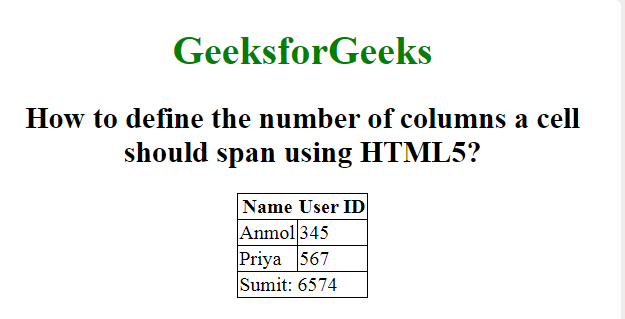Related Articles

# How to define the number of columns a cell should span using HTML5 ?

• Last Updated : 28 Oct, 2020

This article will show you how a column can be made to span over multiple cells. This is done using the colspan attribute when using the <td> tag. This allows the single table cell to span the width of more than one cell or column. It provides similar functionality to the “merge cell” functions in spreadsheet programs like Excel.

Syntax:

`<td colspan = "value">table content...</td>`

Example:

 ```<``html``>`` ` `<``head``>``    ``<``style``>``        ``body {``            ``text-align: center;``        ``}`` ` `        ``h1 {``            ``color: green;``        ``}`` ` `        ``table,``        ``tbody,``        ``td {``            ``border: 1px solid black;``            ``border-collapse: collapse;``        ``}``    `````` ` `<``body``>``    ``<``h1``>GeeksforGeeks`` ` `    ``<``h2``>``        ``How to define the number of``        ``columns a cell should span``        ``using HTML5?``    ```` ` `    ``<``table``>`` ` `        ````        ``<``tr``>``            ``<``th``>Name``            ``<``th``>User ID``        ````        ``<``tr``>``            ``<``td``>Anmol``            ``<``td``>345``        ````        ``<``tr``>``            ``<``td``>Priya``            ``<``td``>567``        ```` ` `        ````        ``<``tr``>``            ````            ``<``td` `colspan``=``"2"``>``                ``Sumit: 6574``            ````        ````    `````` ` ``

Output:Supported Browsers: